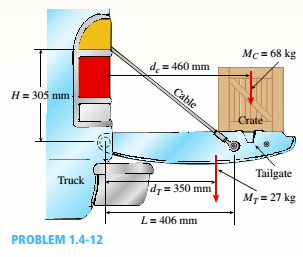# Solve the preceding problem if the mass of the tailgate is M T — 11 kg and that of the crate is hi c — 6S kg. Use dimensions H = 305 mm, L = 406 mm, d c = 460 mm, and d T = 350 mm. The cable cross-sectional area is A t = 11.0 mm'. (a) Find the tensile Force T and normal stress T in each cable. (b) IF each cable elongates &amp; = 0.25 mm due to the weight or both the crate and the tailgate, what is the average strain in the cable?### Mechanics of Materials (MindTap Co...

9th Edition
Barry J. Goodno + 1 other
Publisher: Cengage Learning
ISBN: 9781337093347### Mechanics of Materials (MindTap Co...

9th Edition
Barry J. Goodno + 1 other
Publisher: Cengage Learning
ISBN: 9781337093347
Chapter 1, Problem 1.4.12P
Textbook Problem
194 views

## Solve the preceding problem if the mass of the tailgate is MT— 11 kg and that of the crate is hic— 6S kg. Use dimensions H = 305 mm,L = 406 mm, dc= 460 mm, and dT= 350 mm. The cable cross-sectional area is At= 11.0 mm'.(a) Find the tensile Force T and normal stress T in each cable.(b) IF each cable elongates & = 0.25 mm due to the weight or both the crate and the tailgate, what is the average strain in the cable?(a)

To determine

Tensile force T and normal stress σin each cable.

### Explanation of Solution

Given Information:

You have the following figure with all relevant information:

and

cross -sectional area Ae=11 mm.2

Calculation:

Consider the free body diagram as:

Take equilibrium of moments about pivot point as:

M=02Tsinθ×406=27×350×9.8+68×18×9.8T=27×350×9.8+68×18×9

(b)

To determine

Average strain in the cable.

### Still sussing out bartleby?

Check out a sample textbook solution.

See a sample solution

#### The Solution to Your Study Problems

Bartleby provides explanations to thousands of textbook problems written by our experts, many with advanced degrees!

Get Started

Find more solutions based on key concepts
Explain the major diameter of a thread.

Precision Machining Technology (MindTap Course List)

What is a relational diagram? Give an example.

Database Systems: Design, Implementation, & Management

What are the main components of a database system?

Database Systems: Design, Implementation, & Management

For Problem 19.22, determine the probability (assuming normal distribution) that a light bulb would have a life...

Engineering Fundamentals: An Introduction to Engineering (MindTap Course List)

Explain the differences between a Gantt chart and a PERT/CPM chart

Systems Analysis and Design (Shelly Cashman Series) (MindTap Course List)

Besides the web, what other Internet services are available? (88)

Enhanced Discovering Computers 2017 (Shelly Cashman Series) (MindTap Course List)

Use an elastic analysis and determine the maximum load per inch of weld.

Steel Design (Activate Learning with these NEW titles from Engineering!)

If your motherboard supports ECC DDR3 memory, can you substitute non-ECC DDR3 memory?

A+ Guide to Hardware (Standalone Book) (MindTap Course List)